﻿ Some Types of Integral Problems

Some Types of Integral Problems

Chii-Huei YuOPEN ACCESSPEER-REVIEWED

Some Types of Integral Problems

Department of Management and Information, Nan Jeon University of Science and Technology, Tainan City, Taiwan

Abstract

This paper uses the mathematical software Maple for the auxiliary tool to study four types of integrals. We can obtain the infinite series forms of these integrals by using binomial theorem and integration term by term theorem. In addition, we propose two examples to do calculation practically. The research methods adopted in this study involved finding solutions through manual calculations and verifying these solutions by using Maple.

• Yu, Chii-Huei. "Some Types of Integral Problems." American Journal of Systems and Software 2.1 (2014): 22-26.
• Yu, C. (2014). Some Types of Integral Problems. American Journal of Systems and Software, 2(1), 22-26.
• Yu, Chii-Huei. "Some Types of Integral Problems." American Journal of Systems and Software 2, no. 1 (2014): 22-26.

 Import into BibTeX Import into EndNote Import into RefMan Import into RefWorks

1. Introduction

The computer algebra system (CAS) has been widely employed in mathematical and scientific studies. The rapid computations and the visually appealing graphical interface of the program render creative research possible. Maple possesses significance among mathematical calculation systems and can be considered a leading tool in the CAS field. The superiority of Maple lies in its simple instructions and ease of use, which enable beginners to learn the operating techniques in a short period. In addition, through the numerical and symbolic computations performed by Maple, the logic of thinking can be converted into a series of instructions. The computation results of Maple can be used to modify our previous thinking directions, thereby forming direct and constructive feedback that can aid in improving understanding of problems and cultivating research interests. Inquiring through an online support system provided by Maple or browsing the Maple website (www.maplesoft.com) can facilitate further understanding of Maple and might provide unexpected insights. As for the instructions and operations of Maple, we can refer to [1-7].

In calculus and engineering mathematics courses, we learnt many methods to solve the integral problems including change of variables method, integration by parts method, partial fractions method, trigonometric substitution method, and so on. In this paper, we study the following four types of integrals which are not easy to obtain their answers using the methods mentioned above.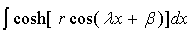(1)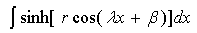(2)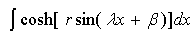(3)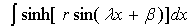(4)

where r,λ,β are real numbers, r,λ≠0. We can obtain the infinite series forms of these integrals by using binomial theorem and integration term by term theorem; these are the major results of this paper (i.e., Theorems 1,2). As for the study of related integral problems can refer to [8-24]. On the other hand, we provide some integrals to do calculation practically. The research methods adopted in this study involved finding solutions through manual calculations and verifying these solutions by using Maple. This type of research method not only allows the discovery of calculation errors, but also helps modify the original directions of thinking from manual and Maple calculations. For this reason, Maple provides insights and guidance regarding problem-solving methods.

2. Main Results

Firstly, we introduce some notations and some formulas used in this study.

2.1. Notations
2.1.1. Suppose m,n are positive integers, mn.

Define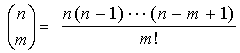for all mn, and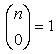.

2.1.2. Assume r is a real number, the largest integer less than or equal to r is denoted by [r].2.2. Formulas
2.2.1. Euler's formula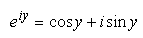, where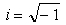, y is any real number.

2.2.2. Taylor series expression of the hyperbolic cosine function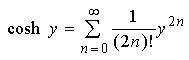, where y is any real number.

2.2.3. Taylor series expression of the hyperbolic sine function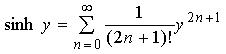, where y is any real number.

Next, we introduce two important theorems used in this paper.

2.3. Theorems
2.3.1. Binomial theorem

Suppose x,y are complex numbers, and n is any positive integer. Then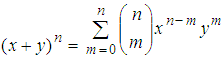.

2.3.2. Integration term by term theorem 

Suppose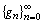is a sequence of Lebesgue integrable functions defined on an interval I. If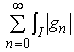is convergent, then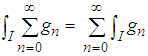.

Before deriving the first major result of this study, we need a lemma.

2.4. Lemma 1

Suppose λ,β are real numbers, λ≠0, n is any positive integer, p is any non-negative integer, and C is a constant. Then the integrals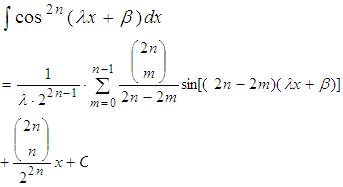(5)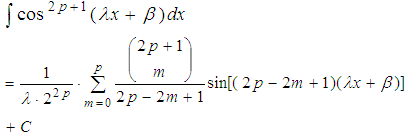(6)

Proof Because for any positive integer k,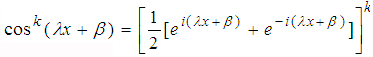(By Euler's formula)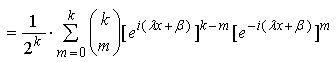(By binomial theorem)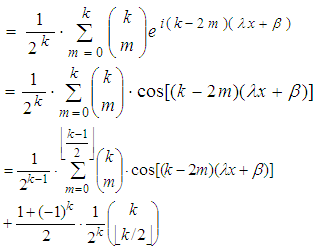(7)

It follows that the integral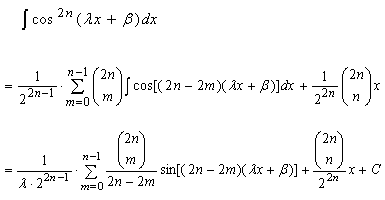And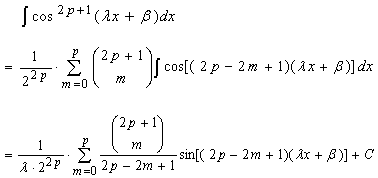In the following, we obtain the infinite series forms of the integrals (1) and (2).

2.5. Theorem 1

Let r,λ,β be real numbers, r,λ≠0.and C is a constant.

(1) The integral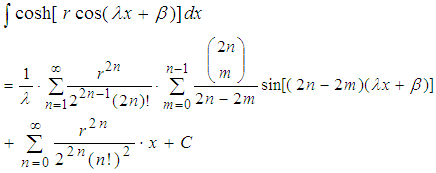(8)

(2) The integral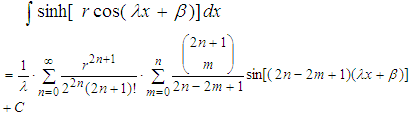(9)

Proof (1) The integral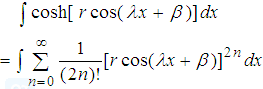(By Formula 2.2.2.)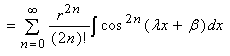(By integration term by term theorem)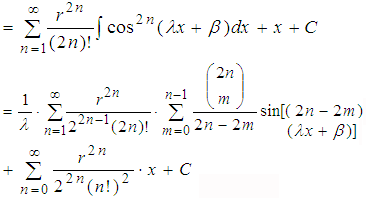(By (5))

(2) The integral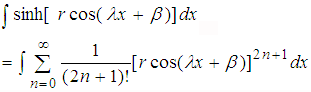(By Formula 2.2.3.)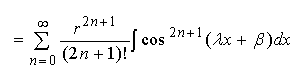(By integration term by term theorem)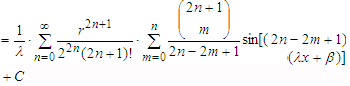(By (6))

To prove the second major result of this study, we also need a lemma.

2.6. Lemma 2

If the assumptions are the same as Lemma 1, then the integrals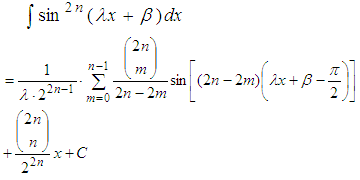(10)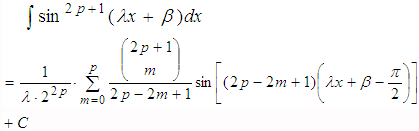(11)

Proof Because for any positive integer k,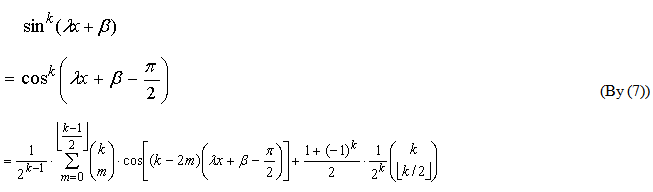It follows that the integral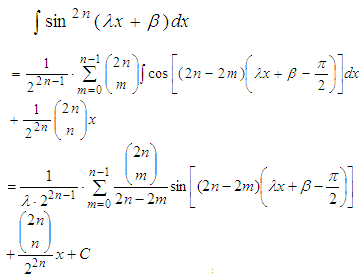And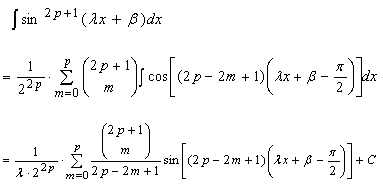Finally, we determine the infinite series forms of the integrals (3) and (4).

2.7. Theorem 2

If the assumptions are the same as Theorem 1.

(1) The integral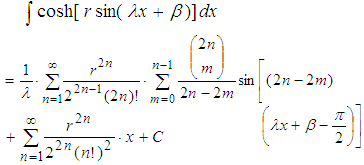(12)

(2) The integral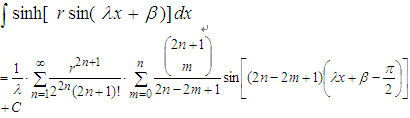(13)

Proof (1) The integral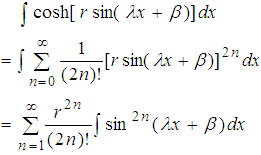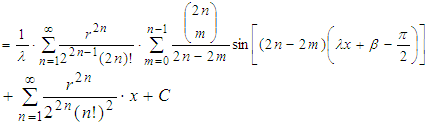By (10)

(2) The integral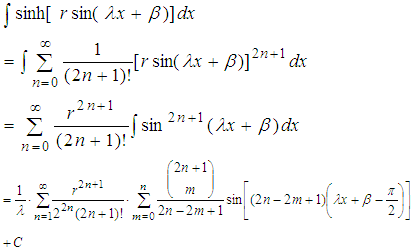By (11)

3. Examples

In the following, for the four types of integral problems in this study, we provide two examples and use Theorems 1,2 to determine the infinite series forms of these integrals. On the other hand, we evaluate some related definite integrals and employ Maple to calculate the approximations of these definite integrals and their solutions for verifying our answers.

3.1. Example 1
3.1.1. Using (8) of Theorem 1, we obtain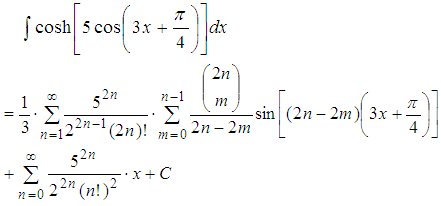(14)

Therefore, we can determine the definite integral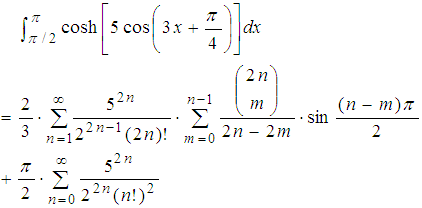(15)

Next, we use Maple to verify the correctness of (15).

>evalf(int(cosh(5*cos(3*x+Pi/4)),x=Pi/2.Pi),18);

54.2832471573584164

>evalf(2/3*sum(5^(2*n)/(2^(2*n-1)*(2*n)!)*sum((2*n)!/(m!*(2*n-m)!*(2*n-2*m))*sin((n-m)*Pi/2),m=0.(n-1)),n=1.infinity)+Pi/2*sum(5^(2*n)/(2^(2*n)*(n!)^2),n=0.infinity),18);

54.2832471573584164

3.1.2. By (9) of Theorem 1, we obtain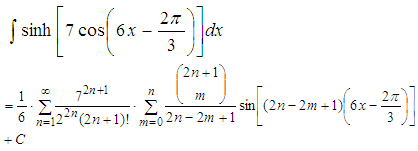(16)

Thus, the definite integral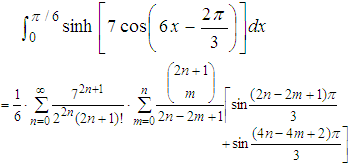(17)

Using Maple to verify the correctness of (17) as follows:

>evalf(int(sinh(7*cos(6*x-2*Pi/3)),x=0.Pi/6),18);

87.4180616135055458

>evalf(1/6*sum(7^(2*n+1)/(2^(2*n)*(2*n+1)!)*sum((2*n+1)!/(m!*(2*n-m+1)!*(2*n-2*m+1))*(sin((2*n-2*m+1)*Pi/3)+sin((4*n-4*m+2)*Pi/3)),m=0.n),n=0.infinity),18);

87.418061635055493 – 5.99593039950345028•10-17I

The above answer obtained by Maple appears I (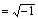), it is because Maple calculates by using special functions built in. The imaginary part is very small, so can be ignored.

3.2. Example 2
3.2.1. By (12) of Theorem 2, we have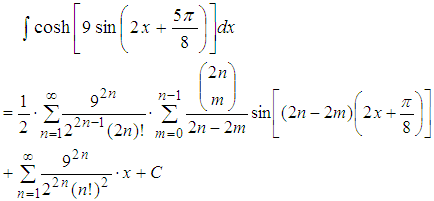(18)

Hence, the definite integral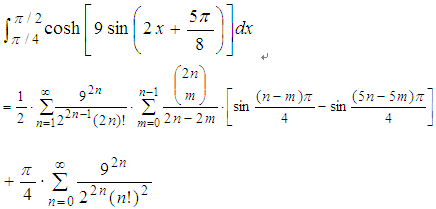(19)

>evalf(int(cosh(9*sin(2*x+5*Pi/8)),x=Pi/4.Pi/2),18);

1503.11190390538857

>evalf(1/2*sum(9^(2*n)/(2^(2*n-1)*(2*n)!)*sum((2*n)!/(m!*(2*n-m)!*(2*n-2*m))*(sin((n-m)*Pi/4)-sin((5*n-5*m)*Pi/4)),m=0.(n-1)),n=1.infinity)+Pi/4*sum(9^(2*n)/(2^(2*n)*(n!)^2),n=0.infinity),18);

1503.11190390538857

3.2.2. Using (13) of Theorem 2, we can determine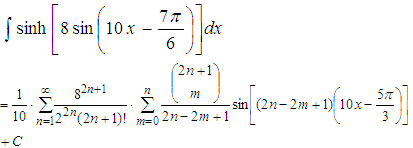(20)

Therefore, the definite integral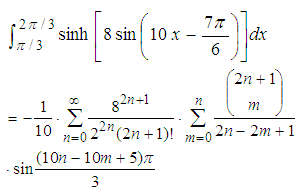(21)

>evalf(int(sinh(8*sin(10*x-7*Pi/6)),x=Pi/3.2*Pi/3),18);

66.7890398786811806

>evalf(-1/10*sum(8^(2*n+1)/(2^(2*n)*(2*n+1)!)*sum((2*n+1)!/(m!*(2*n-m+1)!*(2*n-2*m+1))*sin((10*n-10*m+5)*Pi/3),m=0.n),n=0.infinity),18);

66.789039876811832-6.84850388804298574•10-17I

Also, the imaginary part of the above answer obtained by Maple is very small, so can be ignored.

4. Conclusion

In this paper, we provide a new technique to solve some integrals. We hope this technique can be applied to evaluate another integral problems. On the other hand, the binomial theorem and the integration term by term theorem play significant roles in the theoretical inferences of this study. In fact, the applications of these two theorems are extensive, and can be used to easily solve many difficult problems; we endeavor to conduct further studies on related applications. In addition, Maple also plays a vital assistive role in problem-solving. In the future, we will extend the research topic to other calculus and engineering mathematics problems and solve these problems by using Maple. These results will be used as teaching materials for Maple on education and research to enhance the connotations of calculus and engineering mathematics.

References

  C. T. J. Dodson and E. A. Gonzalez, Experiments in mathematics using Maple, Springer-Verlag, New York, 1995.In article CrossRef  D. Richards Advanced mathematical methods with Maple, Cambridge University Press, New York, 2002.In article  M. L. Abell and J. P. Braselton, Maple by example, 3rd ed., Elsevier Academic Press, New York, 2005.In article  R. J. Stroeker and J. F. Kaashoek, Discovering mathematics with Maple: an interactive exploration for mathematicians, engineers and econometricians, Birkhauser Verlag, Basel, 1999.In article CrossRef  F. Garvan, the Maple book, Chapman & Hall/CRC, London, 2001.In article  J. S. Robertson, Engineering mathematics with Maple, McGraw-Hill, New York, 1996.In article  C. Tocci and S. G. Adams, Applied Maple for engineers and scientists, Artech House, Boston, 1996.In article  C. Oster, “Limit of a definite integral,” SIAM Review, vol. 33, no. 1, pp. 115-116, 1991.In article CrossRef  A. A. Adams, H. Gottliebsen, S. A. Linton, and U. Martin, “Automated theorem proving in support of computer algebra: symbolic definite integration as a case study, ”Proceedings of the 1999 International Symposium on Symbolic and Algebraic Computation, pp. 253-260, Vancouver, Canada, 1999.In article CrossRef  M. A. Nyblom, “On the evaluation of a definite integral involving nested square root functions,” Rocky Mountain Journal of Mathematics, vol. 37, no. 4, pp. 1301-1304, 2007.In article CrossRef  C. -H. Yu, “Using Maple to study two types of integrals,” International Journal of Research in Computer Applications and Robotics, vol. 1, issue. 4, pp. 14-22, 2013.In article  C. -H. Yu, “Solving some integrals with Maple,” International Journal of Research in Aeronautical and Mechanical Engineering, vol. 1, issue. 3, pp. 29-35, 2013.In article  C. -H. Yu, “A study on integral problems by using Maple,” International Journal of Advanced Research in Computer Science and Software Engineering, vol. 3, issue. 7, pp. 41-46, 2013.In article  C. -H. Yu, “Evaluating some integrals with Maple,” International Journal of Computer Science and Mobile Computing, vol. 2, issue. 7, pp. 66-71, 2013.In article  C. -H. Yu, “A study of some integral problems using Maple,” Mathematics and Statistics, vol. 2, no. 1, pp. 1-5, 2014.In article  C.-H. Yu, “Application of Maple on the integral problems,” Applied Mechanics and Materials, vols. 479-480, pp. 849-854, 2013.In article CrossRef  C.-H. Yu, “Application of Maple on the integral problem of some type of rational functions,”Proceedings of the Annual Meeting and Academic Conference for Association of IE, D357-D362, 2012.In article  C.-H. Yu, “Application of Maple on evaluation of definite integrals,” Applied Mechanics and Materials, vols. 479-480, pp. 823-827, 2013.In article CrossRef  C. -H. Yu, “Using Maple to study the integrals of trigonometric functions,” Proceedings of the 6th IEEE/International Conference on Advanced Infocomm Technology, no. 00294, 2013.In article  C.-H. Yu, “Application of Maple on some type of integral problem,” Proceedings of the Ubiquitous-Home Conference 2012, pp. 206-210, 2012.In article  C. -H. Yu, “A study of the integrals of trigonometric functions with Maple,” Proceedings of the Institute of Industrial Engineers Asian Conference 2013, Springer, vol. 1, pp. 603-610, 2013.In article  C.-H. Yu, “Application of Maple on some integral problems,” Proceedings of the International Conference on Safety & Security Management and Engineering Technology 2012, pp. 290-294, 2012.In article  C.-H. Yu, “Application of Maple on evaluating the closed forms of two types of integrals,” Proceedings of the 17th Mobile Computing Workshop, ID 16, 2012.In article  C.-H. Yu, “Application of Maple: taking two special integral problems as examples,” Proceedings of the 8th International Conference on Knowledge Community, pp. 803-811, 2012.In article  Hyperbolic functions, online available from http://en.wikipedia.org/wiki/Hyperbolic_functionIn article  T. M. Apostol, Mathematical analysis, 2nd ed., Addison-Wesley Publishing, Boston, p269, 1975.In article